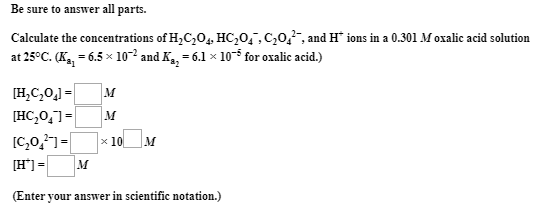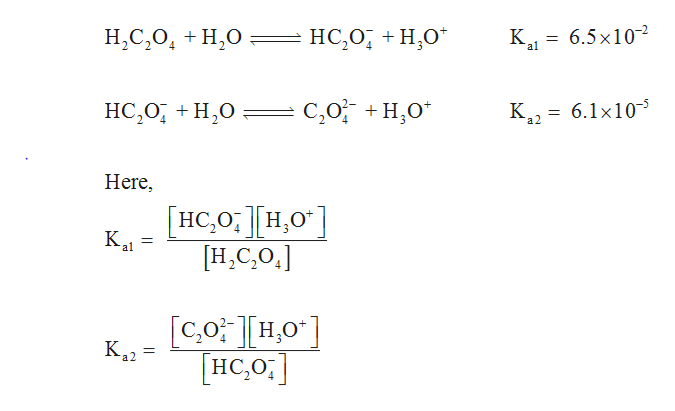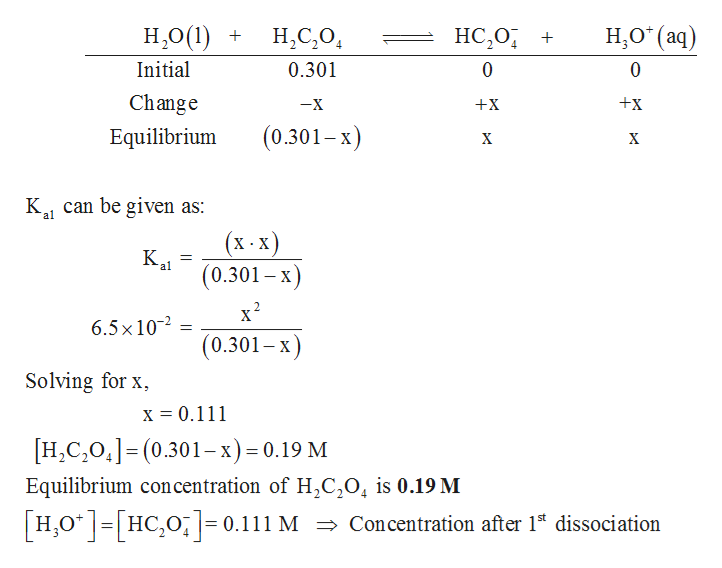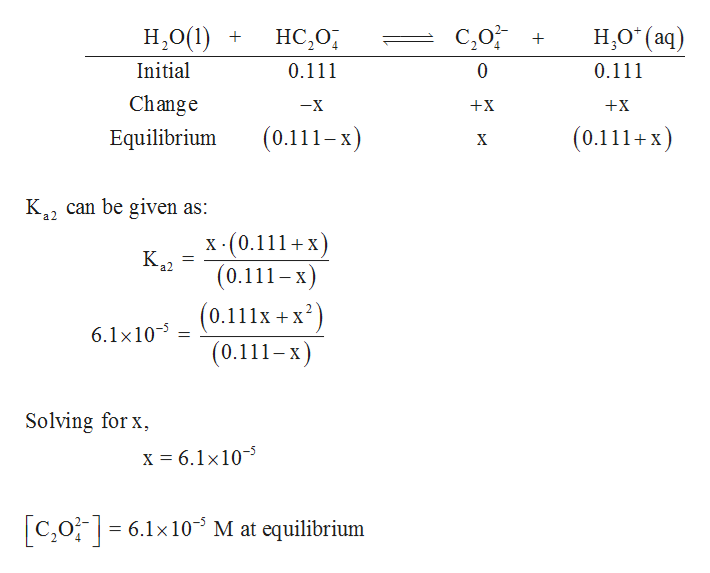# Be sure to answer all parts.Calculate the concentrations of H,C,0,, HC,0,,C,0,, and H* ions in a 0.301 M oxalic acid solutionat 25°C. (K,, = 6.5 x 10-2 and K,, = 6.1 x 105 for oxalic acid.)[H,C,0,] =|м[HC,0,]=[C,0,]=[H'] = Mx 10 M(Enteryour answer in scientific notation.)

Question
1 viewshelp_outlineImage TranscriptioncloseBe sure to answer all parts. Calculate the concentrations of H,C,0,, HC,0,,C,0,, and H* ions in a 0.301 M oxalic acid solution at 25°C. (K,, = 6.5 x 10-2 and K,, = 6.1 x 105 for oxalic acid.) [H,C,0,] = |м [HC,0,]= [C,0,]= [H'] = M x 10 M (Enter your answer in scientific notation.) fullscreen
check_circle

Step 1

Dissociation reactions of oxalic acid is given below,help_outlineImage TranscriptioncloseН.С,О, + Н,о НС,О, + Н,о" K, — 6.5х10-2 al С,о +Н,о" 6.1x10-5 НС,О, + Н,О K, = Here, [нC о: [н,о"] [Н.С.О,] [со: Тно] нC о:] K,2 fullscreen
Step 2

ICE table is constructed for the first dissociation as follows,help_outlineImage TranscriptioncloseН.О (ад H,O(1) + H,C,O, НС 0, + Initial 0.301 Change +x -X +X (0.301- x) Equilibrium X х K, can be given as: (x -X) Kal (0.301 – x) х? 6.5x10-2 (0.301– x Solving for x, X = 0.111 [H,C,0,]=(0.301– x) = 0.19 M Equilibrium concentration of H,C,0, is 0.19 M [H,0*]=[HC,0;]= 0.111 M = Concentration after 1* dissociation fullscreen
Step 3

ICE table is constructed for the sec...help_outlineImage TranscriptioncloseC,0 + Н.О (ад) Н.О(1) + НС,О, Initial 0.111 0.111 Change +X -X +X (0.111+x) (0.111-x) Equilibrium X K, can be given as: X - (0.111+ x) (0.111-x) ra2 (0.111x + x?) 6.1x10- = (0.111-x) Solving for x, X = 6.1x10- C,0;= 6.1x10³ M at equilibrium fullscreen

### Want to see the full answer?

See Solution

#### Want to see this answer and more?

Solutions are written by subject experts who are available 24/7. Questions are typically answered within 1 hour.*

See Solution
*Response times may vary by subject and question.
Tagged in

### Chemistry